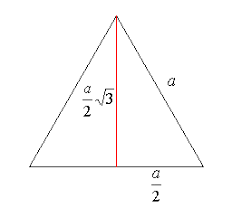# Java Program Perimeter Of Equilateral Triangle | Programs

Java Program to calculate the perimeter of an equilateral triangle – In this particular piece, we will brief in on how to calculate the perimeter of an equilateral triangle.

Suitable examples and sample programs have been added for the better apprehension of the mentioned codes. The compiler has also been added with which you can execute the codes yourself.

The means of calculating the perimeter of an equilateral triangle in Java programming in this article are as follows:

• Using Scanner Class
• Using Command Line Arguments
• Using Static Function

An equilateral triangle, as we all know, is a triangle with all of its sides equal. Owing to the angle-side relationship, all the angles of an equilateral triangle are equal to 60 degrees.

This is how an equilateral triangle looks like:As you can see, this is an equilateral triangle with a side length of “a” units.

The perimeter of an equilateral triangle can be calculated with this formula:

Perimeter = a + a + a = 3a

The altitude of an equilateral triangle bisects the opposite side in equal halves.

Thus, by applying Pythagoras Theorem, we can calculate the height to be [(sqrt3)/2]*a

So, the area of the triangle is:

Area = 1/2 * [(sqrt3)/2]*a * a = [(sqrt 3)/4]*a^2

Hence, the several ways to calculate the perimeter of an equilateral triangle in Java Programming are as follows:

Output:

Output:

Output:

x

## Plus Star Pattern Java Program | Patterns

Java program to print plus star pattern program – We have written the below print/draw ...